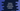# Python program to calculate compound interest## Compound interest calculation in Python :

Compound interest is an interest calculation process that calculates the interest based on the initial principal and accumulated interests on a compounding period. Interest is added to the principal and for the next period, interest is gained on the accumulated interest.

In this post, I will show you how to find the compound interest in python programming with one example.

### Formula to find compound interest :

The below formula is used to find compound interest :

``A = P (1 + R/(100 * n))^nt``

Here, A = The final amount i.e. intial amount + compound interest P = Principal amount or initial amount R = The yearly rate of interest n = Number of compounding periods yearly t = Number of years

### Python program :

``````def findCompoundInterest(P, R, t, n):
return P * pow((1 + R/(100 * n)), n*t);

P = float(input("Enter principal amount : "))
R = float(input("Enter annual rate of interest : "))
t = float(input("Enter time in years : "))
n = float(input("Enter number of compounding periods per year : "))

A = findCompoundInterest(P,R,t,n)

print("Total amount : {}".format(A))
print("Compound interest : {}".format(A-P))``````

findCompoundInterest method is used to find out the total amount including compound interest i.e. A. We are taking the inputs as float from the user and calculating the values.

### Sample output :

``````Enter principal amount : 1000
Enter annual rate of interest : 20
Enter time in years : 10
Enter number of compounding periods per year : 2
Total amount : 6727.499949325611
Compound interest : 5727.499949325611

Enter principal amount : 10000
Enter annual rate of interest : 5
Enter time in years : 10
Enter number of compounding periods per year : 1
Total amount : 16288.94626777442
Compound interest : 6288.9462677744195``````